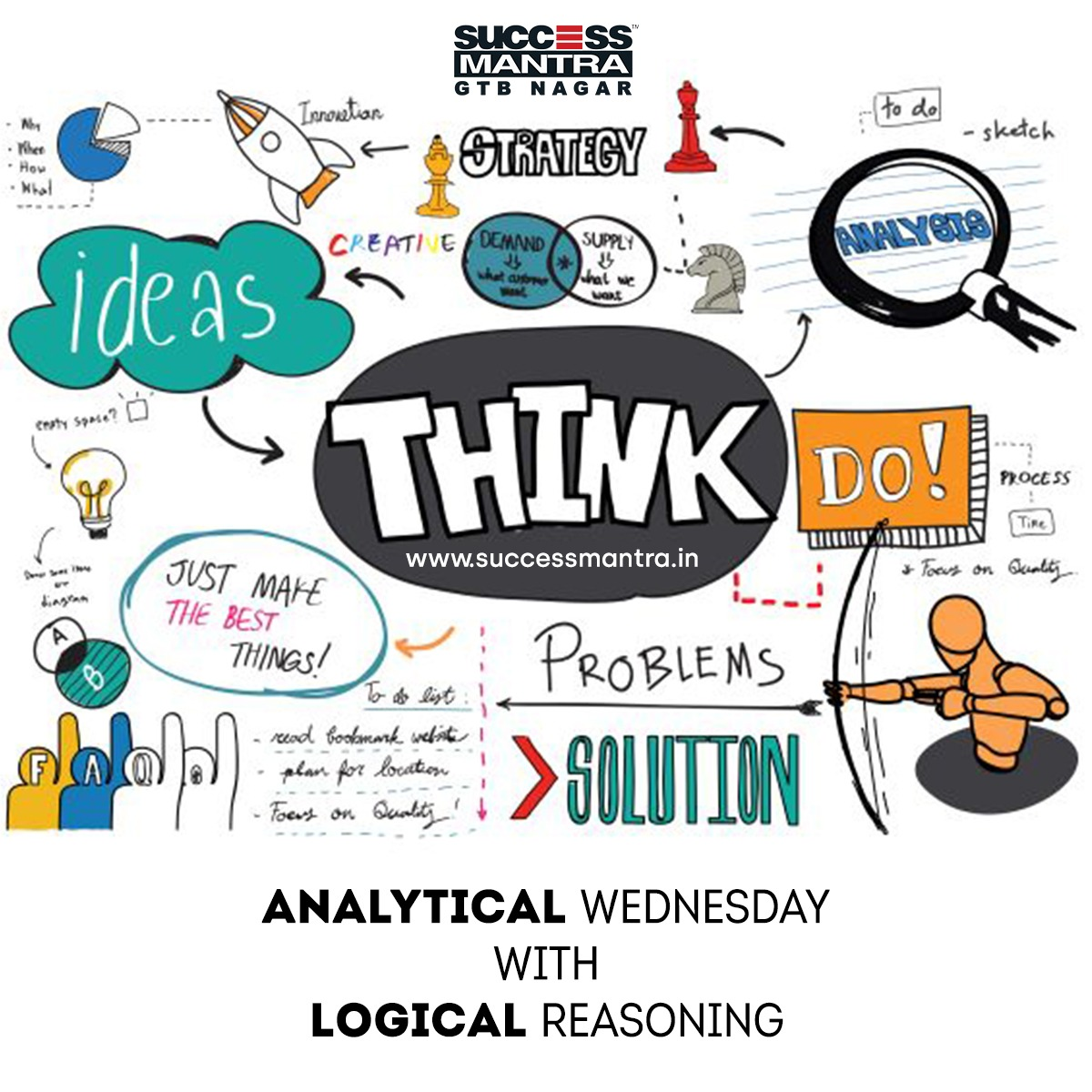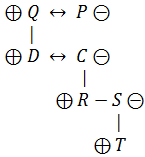## Questions on Logical Reasoning SMLRQ023Directions for Questions 01-05: Answer the questions on the basis of the following Information.

• A%B means A is father of B
• A\$B means B is wife of A
• A*B means B is son of A
• A#B means both are on the same level
• A&B means B is sister of A
• A@B means A is brother of B
• A^B means A is daughter of B

Statement 1:

Q%D#C*R@S*T; P*D; S^D

Question 1. How many male members are there in the family?

A. Two
B. Three
C. Four
D. Five

Question 2. How is P related to Q?

A. Husband
B. Wife
C. Sister
D. Brother

Question 3. How is R related to Q?

A. Grandson
B. Daughter
C. Granddaughter
D. Daughter-in-law

Question 4. How many generations are there in the family?

A. Two
B. Three
C. Four
D. Five

Question 5. How is C related to P?

A. Grandson
B. Daughter
C. Granddaughter
D. Daughter-in-law